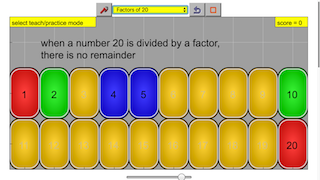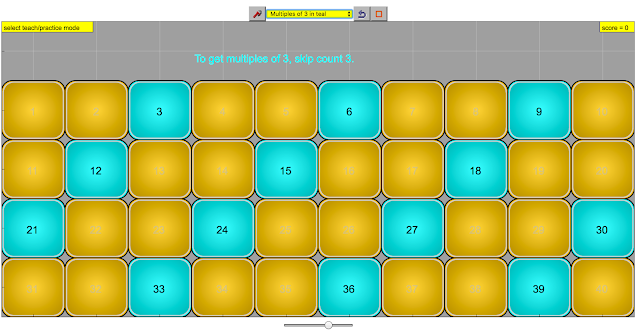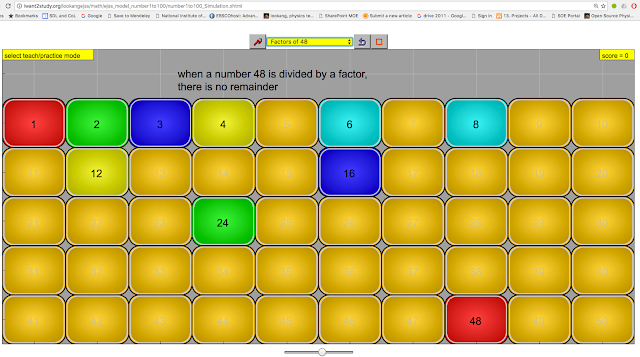### Translations

Code Language Translator Run### Software Requirements

SoftwareRequirements

 Android iOS Windows MacOS with best with Chrome Chrome Chrome Chrome support full-screen? Yes. Chrome/Opera No. Firefox/ Samsung Internet Not yet Yes Yes cannot work on some mobile browser that don't understand JavaScript such as..... cannot work on Internet Explorer 9 and below

### CreditsThis email address is being protected from spambots. You need JavaScript enabled to view it.; Francisco Esquembre; Felix J. Garcia Clemente

## Apps

### Sample Learning Goals

Topics
Primary 3
1 WHOLE NUMBERS
Numbers up to 10 000
Include:
• number notation and place values (thousands, hundreds, tens, ones),
• reading and writing numbers in numerals and in words,
• comparing and ordering numbers,
• odd and even numbers,
• number patterns

Primary 4
1 Whole Numbers
Factors and multiples
Include:
• determining if a 1-digit number is a factor of a given number,
• listing all factors of a given number up to 100,
• finding the common factors of two given numbers,
• recognising the relationship between factor and multiple,
• determining if a number is a multiple of a given 1-digit number,
• listing the first 12 multiples of a given 1-digit number,
• finding the common multiples of two given 1-digit numbers.
Exclude 'highest common factor' (H.C.F.) and 'lowest common multiple’ (L.C.M.).

Primary 5 Foundation Mathematics (Calculator is allowed unless otherwise stated)
1 Whole Numbers
Factors and multiples
Include:
• determining if a 1-digit number is a factor of a given number,
• listing all factors of a given number up to 100,
• finding the common factors of two given numbers,
• recognising the relationship between factor and multiple,
• determining if a number is a multiple of a given 1-digit number,
• listing the first 12 multiples of a given 1-digit number,
• finding the common multiples of two given numbers up to 12.
Exclude 'highest common factor' (H.C.F.) and 'lowest common multiple’ (L.C.M.).

### Research

Teaching Tips

even numbers are easier to understand, ask pupils to line up in pairs, so if it is possible to pair up, the number of pupils is even.

odd numbers is the opposite of even, again, ask pupils to line up in pairs, so if it is not possible to pair up, meaning one pupil has no partner,  the number of pupils is odd.

A Poem on Even & Odd Numbers by Marg Wadsworth

If you are an even number
You always have a pair
So if you look around
Your buddy will always be there
But ...
If you are an odd number
There's always a lonely one
He looks around to find his buddy
But he's the only one.

10 Odd Todd and Even Steven swerdy45

multiples is easier if it is associated to skip count, so mulitples of three is skip count three, for example, 3 ,6, 9, 12, ....multiples of three, skip count three, color coded for ease of pattern recognition

factors is the hardest, pairing the first and the last numbers of the factors could help pupils realisefactors of a numbers colour coded for ease of pattern recognition 1x48 = 2x24 = 3x16 = 4x12 = 6x8 = 48

[text]

### Version:

1. http://weelookang.blogspot.sg/2016/06/numbers-1-to-100-properties.html JavaScript version
2. based on an earlier  swf file from Thong  , Thong's files